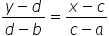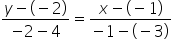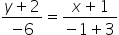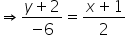Maths-
General
Easy

Question

# Write equation of the line containing (-3, 4) and (-1, -2)Hint:

## The correct answer is: 3x + y + 5=0

### Step by step solution:Let the given points be denoted by(a, b) = (-3, 4)(c, d) = (-1, -2)The equation of a line passing through two points (a, b) and (c, d) isUsing the above points, we haveSimplifying the above equation, we haveCross multiplying, we get2(y + 2) = -6(x + 1)Expanding the factors, we have2y + 4 = -6x - 6Taking all the terms in the left hand side, we have6x + 2y + 4 + 6 = 0Finally, the equation of the line is6x + 2y + 10 = 0Dividing the equation throughout by2, we get3x + y + 5 = 0This is the general form of the equation.This is also the required equation.

We can simplify the equation in any other way and we would still reach the same equation. The general form of an equation in two variables is given by ax + by + c = 0, where a, b, c are real numbers. The student is advised to remember all the different forms of a line, like, slope-intercept form, axis-intercept form, etc.#### With Turito Foundation.#### Get an Expert Advice From Turito.